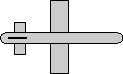# Maths - Quaternion to AxisAngle

## Prerequisites

Definition of terms:

## Equations

angle = 2 * acos(qw)
x = qx / sqrt(1-qw*qw)
y = qy / sqrt(1-qw*qw)
z = qz / sqrt(1-qw*qw)

## Singularities

Axis angle has two singularities at angle = 0 degrees and angle = 180 degrees, so I think that it is a good precaution to check that that the above formula works in these cases. At 0 degrees the axis is arbitrary (any axis will produce the same result), at 180 degrees the axis is still relevant so we have to calculate it.

As explained here the formula for quaternoin in terms of axis angle is:

q = cos(angle/2) + i ( x * sin(angle/2)) + j (y * sin(angle/2)) + k ( z * sin(angle/2))

### at angle = 0 degrees

q = 1 + i 0 + j 0 + k 0

so working back from above equation qw = 1 so :

angle = 2 * acos(qw) = 0
x = qx / sqrt(1-qw*qw) = divide by zero = infinity
y = qy / sqrt(1-qw*qw) = divide by zero = infinity
z = qz / sqrt(1-qw*qw) = divide by zero = infinity

So we have to test for divide by zero, but this is not a problem since the axis can be set to any arbitary value provided that it is normalised.

### at angle = 180 degrees

q = 0 + i x + j y + k z

so working back from above equation qw = 0 so :

angle = 2 * acos(qw) = 2 * 90 degrees = 180 degrees (or -180 degrees which is equivalent)
x = qx / sqrt(1-qw*qw) = qx
y = qy / sqrt(1-qw*qw) = qy
z = qz / sqrt(1-qw*qw) = qw

Which is correct so the formula works properly in this case. Although some axis angle calculations can jump suddenly around 180 degrees, this quaternion to axis-angle translation seems quite smooth at this region.

## Code

Java code to do conversion:

``` public void set(Quat4d q1) {
if (q1.w > 1) q1.normalise(); // if w>1 acos and sqrt will produce errors, this cant happen if quaternion is normalised
angle = 2 * Math.acos(q1.w);
double s = Math.sqrt(1-q1.w*q1.w); // assuming quaternion normalised then w is less than 1, so term always positive.
if (s < 0.001) { // test to avoid divide by zero, s is always positive due to sqrt
// if s close to zero then direction of axis not important
x = q1.x; // if it is important that axis is normalised then replace with x=1; y=z=0;
y = q1.y;
z = q1.z;
} else {
x = q1.x / s; // normalise axis
y = q1.y / s;
z = q1.z / s;
}
}```

## Derivation of Equations

from AxisAngle to Quaternion page we have:

1. --- q1.x = a1.x * Math.sin(a1.angle/2)
2. --- q1.y = a1.y * Math.sin(a1.angle/2)
3. --- q1.z = a1.z * Math.sin(a1.angle/2)
4. --- q1.w = Math.cos(a1.angle/2)

therefore from 4.

a1.angle/2 = Math.acos(q1.w)

5. --- a1.angle = 2 * Math.acos(q1.w)

and from 1.

a1.x = q1.x / Math.sin(a1.angle/2)

substituting from 5 gives:

a1.x = q1.x / Math.sin(Math.acos(q1.w))from above diagram this gives:

a1.x = q1.x / Math.sqrt(1-q1.w*q1.w)

simarly for y and z:

a1.y = q1.y / Math.sqrt(1-q1.w*q1.w)

a1.z = q1.z / Math.sqrt(1-q1.w*q1.w)

## Example

 we take the 90 degree rotation from this:to this:As shown here the quaternion for this rotation is: (0.7071+ i 0.7071)

So using the above result:

angle = 2 * acos(qw) = 2 * acos(0.7071) = 90 degrees
s = sqrt(1-qw*qw) = sqrt(0.5) = 0.7071
x = qx / s = 0.7071 / 0.7071 = 1
y = qy / s = 0
z = qz / s = 0

this gives the axis angle:

angle = 90 degrees
axis = 1,0,0

which agrees with the result shown here

Note:

• Most maths libraries use radians instead of degrees (apart from OpenGL).

## Angle Calculator and Further examples

I have put a java applet here which allows the values to be entered and the converted values shown along with a graphical representation of the orientation.

Also further examples in 90 degree steps here

 metadata block see also: Correspondence about this page Book Shop - Further reading. Where I can, I have put links to Amazon for books that are relevant to the subject, click on the appropriate country flag to get more details of the book or to buy it from them.3D Math Primer - Aimed at complete beginners to vector and matrix algebra.

This site may have errors. Don't use for critical systems.